JEE  >  BITSAT Physics Test - 7

# BITSAT Physics Test - 7

Test Description

## 40 Questions MCQ Test BITSAT Mock Tests Series & Past Year Papers | BITSAT Physics Test - 7

BITSAT Physics Test - 7 for JEE 2023 is part of BITSAT Mock Tests Series & Past Year Papers preparation. The BITSAT Physics Test - 7 questions and answers have been prepared according to the JEE exam syllabus.The BITSAT Physics Test - 7 MCQs are made for JEE 2023 Exam. Find important definitions, questions, notes, meanings, examples, exercises, MCQs and online tests for BITSAT Physics Test - 7 below.
Solutions of BITSAT Physics Test - 7 questions in English are available as part of our BITSAT Mock Tests Series & Past Year Papers for JEE & BITSAT Physics Test - 7 solutions in Hindi for BITSAT Mock Tests Series & Past Year Papers course. Download more important topics, notes, lectures and mock test series for JEE Exam by signing up for free. Attempt BITSAT Physics Test - 7 | 40 questions in 50 minutes | Mock test for JEE preparation | Free important questions MCQ to study BITSAT Mock Tests Series & Past Year Papers for JEE Exam | Download free PDF with solutions
 1 Crore+ students have signed up on EduRev. Have you?
BITSAT Physics Test - 7 - Question 1

### Work of electric motor is

BITSAT Physics Test - 7 - Question 2

### Plutonium decays within half-life of 24000 years. If the plutonium is stored for 72000 years, then the fraction of plutonium that remains is

BITSAT Physics Test - 7 - Question 3

BITSAT Physics Test - 7 - Question 4

EMF is most closely related to

BITSAT Physics Test - 7 - Question 5

A photon of energy 4 eV is incident on a metal surface whose work function is 2 eV. Minimum value of reverse potential to be applied for stopping the emission of electrons is

BITSAT Physics Test - 7 - Question 6

Velocity ratio of the two cathode rays is 1:2. They are applied to the same electric field. What is the deflection ratio of the two cathode rays ?

BITSAT Physics Test - 7 - Question 7

In an L-R circuit, time constant is that time in which current grows from zero to the value

BITSAT Physics Test - 7 - Question 8

A capacitor of capacitance 1 μ F is charged to 1KV. The energy stored in the capacitor in joules is

Detailed Solution for BITSAT Physics Test - 7 - Question 8

C = 1μF
V = 1KV
energy stored U = 1/2 (CV2)
= 1/2* 1*12 = 0.5

BITSAT Physics Test - 7 - Question 9

A sheet of aluminium foil of negligible thickness is introduced between the plates of a capacitor. The capacitance of the capacitor

BITSAT Physics Test - 7 - Question 10

A uniform chain of length L changes partly from a table which is kept in equilibrium by friction. The maximum length that can Withstand without slipping is l, then coefficient of friction between the table and the chain is

BITSAT Physics Test - 7 - Question 11

If R is the radius of a planet, g is the acceleration due to gravity, then the mean density of the planet is given by

BITSAT Physics Test - 7 - Question 12

A body cools from 60oC to 50oC in 10 min. If the room temperature is 25oC and assuming Newton's law of cooling holds good, then temperature of the body after the next 10 min will be

Detailed Solution for BITSAT Physics Test - 7 - Question 12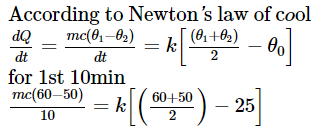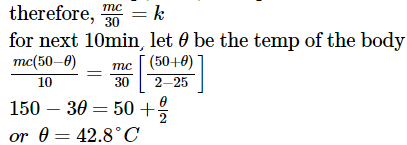BITSAT Physics Test - 7 - Question 13

The kinetic energy of translation of 20 gm of oxygen at 47oC is (molecular wt. of oxygen is 32 gm/mol and R = 8.3 J/mol/K)

BITSAT Physics Test - 7 - Question 14

To convert a galvanometer into a voltmeter, we must connect a

BITSAT Physics Test - 7 - Question 15

To shield an instrument from external magnetic field, it is placed inside a cabin made of

BITSAT Physics Test - 7 - Question 16

The surface tension of a liquid decreases with rise in

BITSAT Physics Test - 7 - Question 17

The value of internal resistance of ideal cell is

BITSAT Physics Test - 7 - Question 18

If an object of weight W and density ρ is submerged in a fluid of density ρ₁, then apparent weight of the object will be

BITSAT Physics Test - 7 - Question 19

Which of the following affets the elasticity of a substance?

BITSAT Physics Test - 7 - Question 20

A car moves along a straight line whose motion is given by : s= 12t+ 3t2 − 2t2, where (s) is in metres and (t) is in seconds. The velocity of the car at start will be

BITSAT Physics Test - 7 - Question 21

The parametric equations of a curve traced by a projectile on a certain planet with no surrounding are given by :y = (8t-5t2) metre and x = 6t metre, where t is in seconds. The velocity with which the projectile is projected, is

BITSAT Physics Test - 7 - Question 22

Which of the following does not represent a zero vector?

BITSAT Physics Test - 7 - Question 23

Consider an elevator moving downwards with an accerleration a, the force exerted by a passenger of mass m on the floor of elevator is

BITSAT Physics Test - 7 - Question 24

In a camera, f-number represents

BITSAT Physics Test - 7 - Question 25

When the displacement is half of the amplitude, then what fraction of the total energy of a simple harmonic oscillator is kinetic ?

BITSAT Physics Test - 7 - Question 26

A coin is placed on a horizontal platform, which undergoes vertical simple harmonic motion of angular frequency ω. The amplitude of oscillations is gradually increased. The coin will have contact with the platform for the first time. (i) at the higher position of the platform (ii) at the mean position of the patform (iii) for an amplitude of g/ω2 (iv) for an amplitude of √2/ω

BITSAT Physics Test - 7 - Question 27

A ray of light is incident on the hypotenuse of a right-angled prism after travelling parallel to the base inside the prism. If ' μ ' is the refractive index of the material of the prism, the maximum value of the base angle for which light is totally reflected from the hypotenuse is

BITSAT Physics Test - 7 - Question 28

A wheel rotates with a constant acceleration of 2.0 radian/sec2. If the wheel starts from rest the number of revolutions it makes in the first ten seconds will be approximately

BITSAT Physics Test - 7 - Question 29

The least coefficient of friction for an inclined plane inclined at angle α with horizontal in order that a solid cylinder will roll down it without slipping is

Detailed Solution for BITSAT Physics Test - 7 - Question 29

Explanation : Acceleration of point A must be zero due to pure rolling.

Thus  mgsinα − f = 0        for point A

mgsinα − μmg cosα = 0

⟹ μ = tanα

BITSAT Physics Test - 7 - Question 30

A thermoelectric refrigerator works on

BITSAT Physics Test - 7 - Question 31

50 g of copper is heated to increase its temprature by 10oC. If the same quantity of heat is given to 10 g water, the rise in its temperature is (Specific heat of copper = 420 Joule -kg-1oC-1. Specific heat of water = 4200 Joule -kg-1oC-1

Detailed Solution for BITSAT Physics Test - 7 - Question 31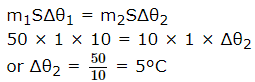BITSAT Physics Test - 7 - Question 32

What is the temperature of source in Carnot engine of 20% efficiency when heat exhausts at 27oC?

BITSAT Physics Test - 7 - Question 33

A stone is tied up to the end of a string and whirled in a vertical circle. The tension in the string is T1 when the stone is at the highest point and T2 when it is at the lowest point. Then

BITSAT Physics Test - 7 - Question 34

From following pairs, choose the pair that does not have identical dimensions

BITSAT Physics Test - 7 - Question 35

In Young's experiment of double slit, the intensity of central bright band is ---- times the individual intensities of the interfering waves

BITSAT Physics Test - 7 - Question 36

The number of beats produced per second by the vibrations x₁ = a sin 320 π t and x₂ = a sin 326 π t is

BITSAT Physics Test - 7 - Question 37

If the two slits in Young's experiment have width ratio 1:4, the ratio of intensity at maxima and minima in the interference pattern in

Detailed Solution for BITSAT Physics Test - 7 - Question 37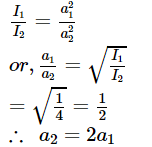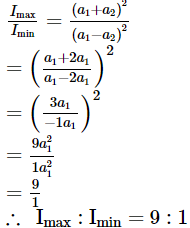BITSAT Physics Test - 7 - Question 38

What will be the wave velocity, if the radar gives 54 waves per min and wavelength of the given wave is 10 m?

BITSAT Physics Test - 7 - Question 39

A bomb of mass 9 kg explodes into 2 pieces of mass 3 kg and 6 kg. The velocity of mass 3 kg is 1-6 m/s, the K.E. of mass 6 kg is

BITSAT Physics Test - 7 - Question 40

4 m3 of water is to be pumped to a height of 20 m and forced into a reservoir at a pressure of 2*105 N/m2 . The work done by the motor is (external pressure = 105 N/m2)

## BITSAT Mock Tests Series & Past Year Papers

2 videos|15 docs|70 tests
 Use Code STAYHOME200 and get INR 200 additional OFF Use Coupon Code
Information about BITSAT Physics Test - 7 Page
In this test you can find the Exam questions for BITSAT Physics Test - 7 solved & explained in the simplest way possible. Besides giving Questions and answers for BITSAT Physics Test - 7, EduRev gives you an ample number of Online tests for practice

## BITSAT Mock Tests Series & Past Year Papers

2 videos|15 docs|70 tests